Courses

# Very Short Answer Type Questions- Circles Class 10 Notes | EduRev

## Class 10 : Very Short Answer Type Questions- Circles Class 10 Notes | EduRev

The document Very Short Answer Type Questions- Circles Class 10 Notes | EduRev is a part of the Class 10 Course Mathematics (Maths) Class 10.
All you need of Class 10 at this link: Class 10

Q1. In the adjoining figure, PA and PB are tangents from P to a circle with centre C. If ∠APB = 40° then find ∠ACB.

Sol. Since a tangent to a circle is perpendicular to the radius through the point of contact,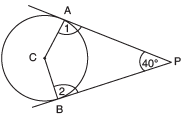∴ ∠1 = 90° and ∠2 = 90°
Now, in quadrilateral APBC, we have:
∠1 + ∠ACB + ∠2 + ∠P = 360°
⇒90° + ∠ACB + 90° + 40°= 360°
⇒∠ACB + 220° = 360°
⇒∠ACB = 360° − 220°
= 140°.

Q2. In the given figure, PA and PB are tangents from P to a circle with centre O. If ∠AOB = 130°, then find ∠APB.

Sol. Since a tangent to a circle is perpendicular to the radius through the point of contact,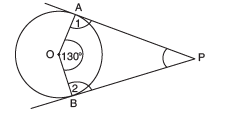∴∠1= ∠2 = 90°
Now, in quadrilateral AOBP, we have:
∠1 + ∠AOB + ∠2 + ∠APB = 360°
⇒ 90° + 130° + 90° + ∠APB = 360°
⇒ 310° + ∠APB = 360°
⇒∠APB = 360 − 310
= 50°
Thus, ∠APB = 50°.

Q3. In the given figure, PT is a tangent to a circle whose centre is O. If PT = 12 cm and PO = 13 cm then find the radius of the circle.

Sol. Since a tangent to a circle is perpendicular to the radius through the point of contact,
∴∠OTP =90°
In rt Δ OTP, using Pythagoras theorem, we get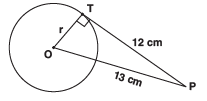OP2 = OT2 + PT2
⇒ 132 = OT2 + 122
⇒ OT2 = 132 − 122 = (13 − 12)
(13 + 12) = 1 × 25 = 25
∴ OT2 = 52
⇒ OT = 5
Thus, radius (r) = 5 cm.

Q4. In the given figure, PT is a tangent to the circle and O is its centre. Find OP.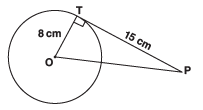Sol. Since, a tangent to a circle is perpendicular to the radius through the point of contact.
∴∠OTP = 90°
In right Δ OTP, using Pythagoras theorem, we get
OP2 = OT2 + PT2
= 82 + 152 = 64 + 225 = 289 = 172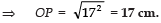Q5. If O is the centre of the circle, then find the length of the tangent AB in the given figure.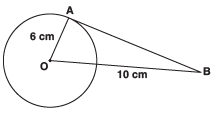Sol. ∵ A tangent to a circle is perpendicular to the radius through the point of contact.
∴ ∠OAB = 90°
Now, in right Δ OAB, we have
OB2 = OA2 + AB2
⇒ 102 = 62 + AB2
⇒ AB2 = 102 − 62 = (10 − 6) (10 +6) = 4 × 16 = 64 = 82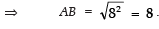Q6. In the figure, PA is a tangent from an external point P to a circle with centre O. If ∠POB = 115° then find ∠APO.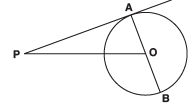Sol. Here, PA is a tangent and OA is radius.
Also, a radius through the point of contact is perpendicular to the tangent.
∴ OA = PA
⇒∠PAO = 90°
In ∆OAP, ∠POB is an external angle,
∴∠APO + ∠PAO = ∠POB
⇒∠ APO + 90° = 115°
⇒ ∠APO = 115° − 90° = 25°

Q7. In the following figure, PA and PB are tangents drawn from a point P to the circle with centre O. If ∠APB = 60°, then what is ∠AOB?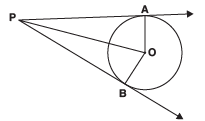Sol. The radius of the circle through the point of contact is perpendicular to the tangent.

∴ OA ⊥ AP and OB ⊥ BP
⇒ ∠PAO = ∠PBO = 90°
∠OAP + ∠APB + ∠PBO + ∠AOB = 360°
90° + 60° + 90° + ∠AOB = 360°
⇒ ∠AOB + 240° = 360°
⇒∠AOB = 360° – 240°
= 120°

Q8. In the figure, CP and CQ are tangents to a circle with centre O. ARB is another tangent touching the circle at R. If QC = 11 cm, BC = 7 cm then find, the length of BR.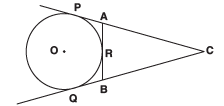Sol. ∵ Tangents drawn from an external point are equal,
∴ BQ = BR ...(1)
And CQ = CP
Since, BC + BQ = QC
⇒ 7 + BR =11 [∵ BQ = BR]
BR = 11 − 7 = 4 cm.

Q9. In the figure, ΔABC is circumscribing a circle. Find the length of BC.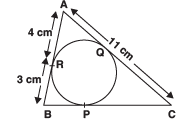Sol. Since tangents drawn from an external point to the circle are equal,
∴ AR = AQ = 4 cm ...(1)
BR = BP = 3 cm ...(2)
PC = QC ...(3)
∴ QC = AC − AQ
= 11 − 4 = 7 cm [From (1)]
BC = BP + PC [From (3)]
= 3 + QC
= (3 + 7) cm = 10 cm

Q10. In the figure, if ∠ ATO = 40°, find ∠ AOB.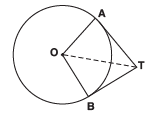Sol. Since the tangent is perpendicular to the radius through the point of contact,
∴ ∠1= ∠4 = 90°
Also, OA = OB    [Radii of the same circle]
OT = OT      [Common]
∴∆OAT ≅∆OBT      [RHS congruency]
⇒∠3= ∠2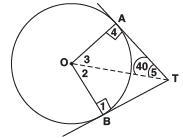Now, in ΔOAT,
∠3 + ∠4 + ∠5 = 180°
⇒ ∠3 + 90° + 40° = 180°
⇒ ∠3 = 180° − 90° − 40° = 50°
⇒ ∠AOB = 50° + 50° = 100°.

Q11. From a point P, the length of the tangent to a circle is 15 cm and distance of P from the centre of the circle is 17 cm, then what is the radius of the circle?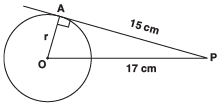Sol. Since radius is perpendicular to the tangent through the point of contact,
∴ OA ⊥ AP
⇒ ∠OAP = 90°
In rt ∆OAP, we have:
OA2 + AP2 = OP2
⇒ r2 + (15)2 = (17)2
r2 = 172 − 152 = (17 − 15) (17 + 15)
= 2 × 32 = 64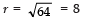Q12. The two tangents from an external point P to a circle with centre O are PA and PB. If ∠APB = 70°, then what is the value of ∠AOB?

Sol. Since tangent is perpendicular to the radius through the point of contact.

∴∠1= ∠2 = 90°
∠AOB + ∠1 + ∠2 + ∠APB = 360°
∠AOB + 90° + 90° + 70° = 360°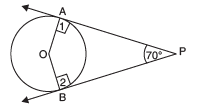⇒∠AOB + 250° = 360°
⇒∠AOB = 360°−250°
= 110°

Offer running on EduRev: Apply code STAYHOME200 to get INR 200 off on our premium plan EduRev Infinity!

,

,

,

,

,

,

,

,

,

,

,

,

,

,

,

,

,

,

,

,

,

;